# RD Sharma Solutions for Class 11 Chapter 28 - Introduction to Three Dimensional Coordinate Geometry Exercise 28.3

In Exercise 28.3 of Chapter 28, we shall discuss some problems related to section formulae. From the exam point of view, solutions are formulated by our subject expert faculty team that contain explanations in a simple language, which is very helpful for students to understand the problems. Students can improve their problem-solving ability by practising the problems provided in the RD Sharma Class 11 Maths Solutions which is very beneficial during their exam preparation. Students can download the pdf from the available links given below.

## Download the pdf of RD Sharma Solutions for Class 11 Maths Exercise 28.3 Chapter 28 – Introduction to Three Dimensional Coordinate Geometry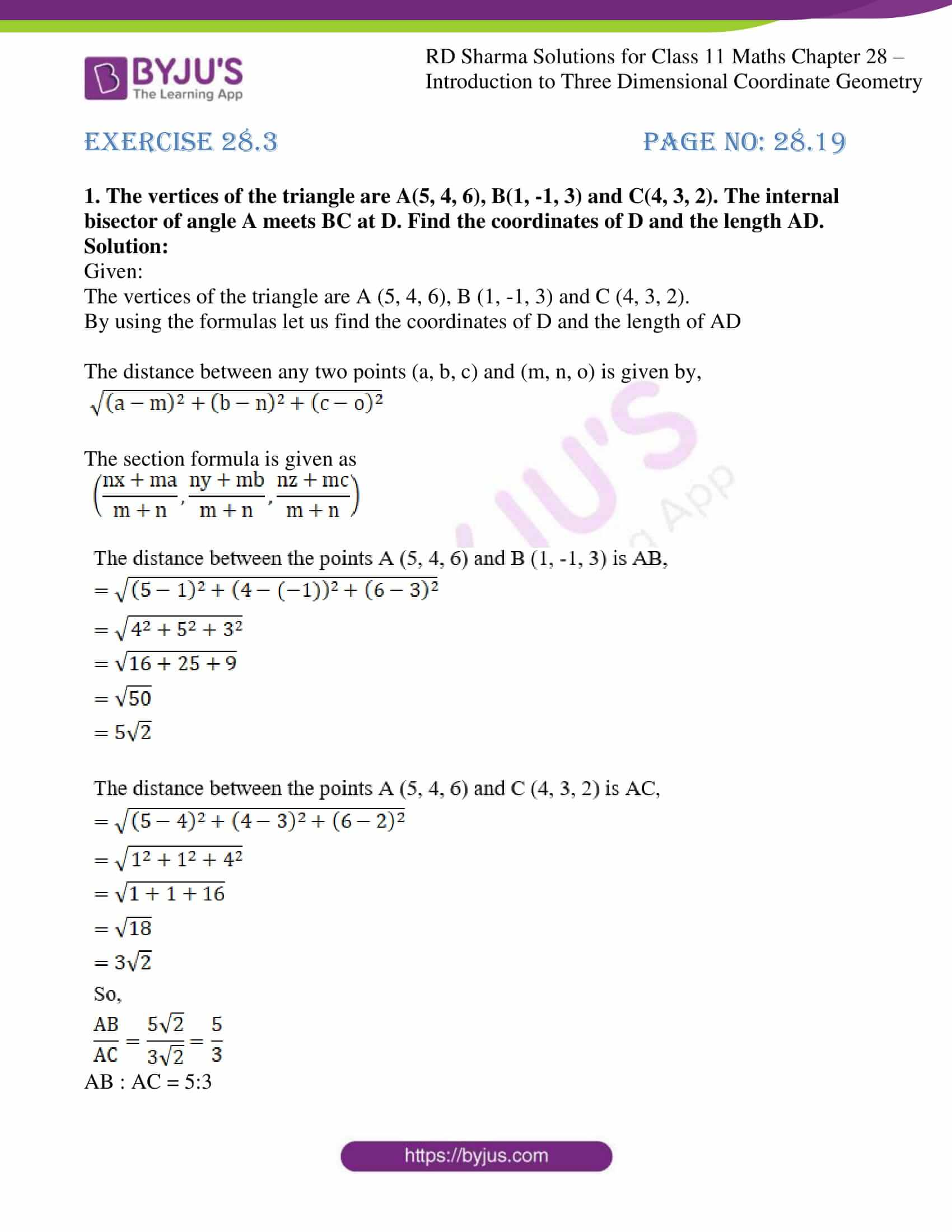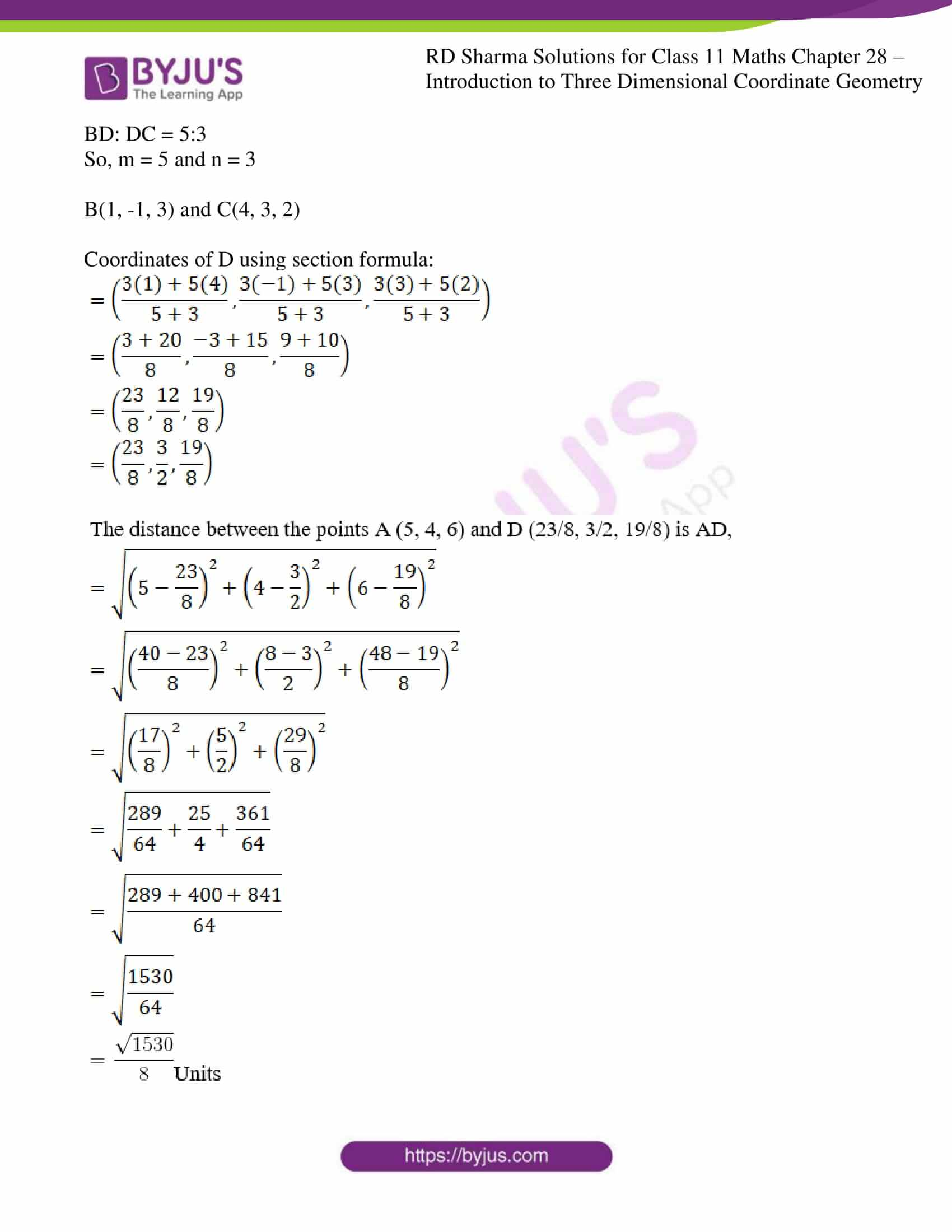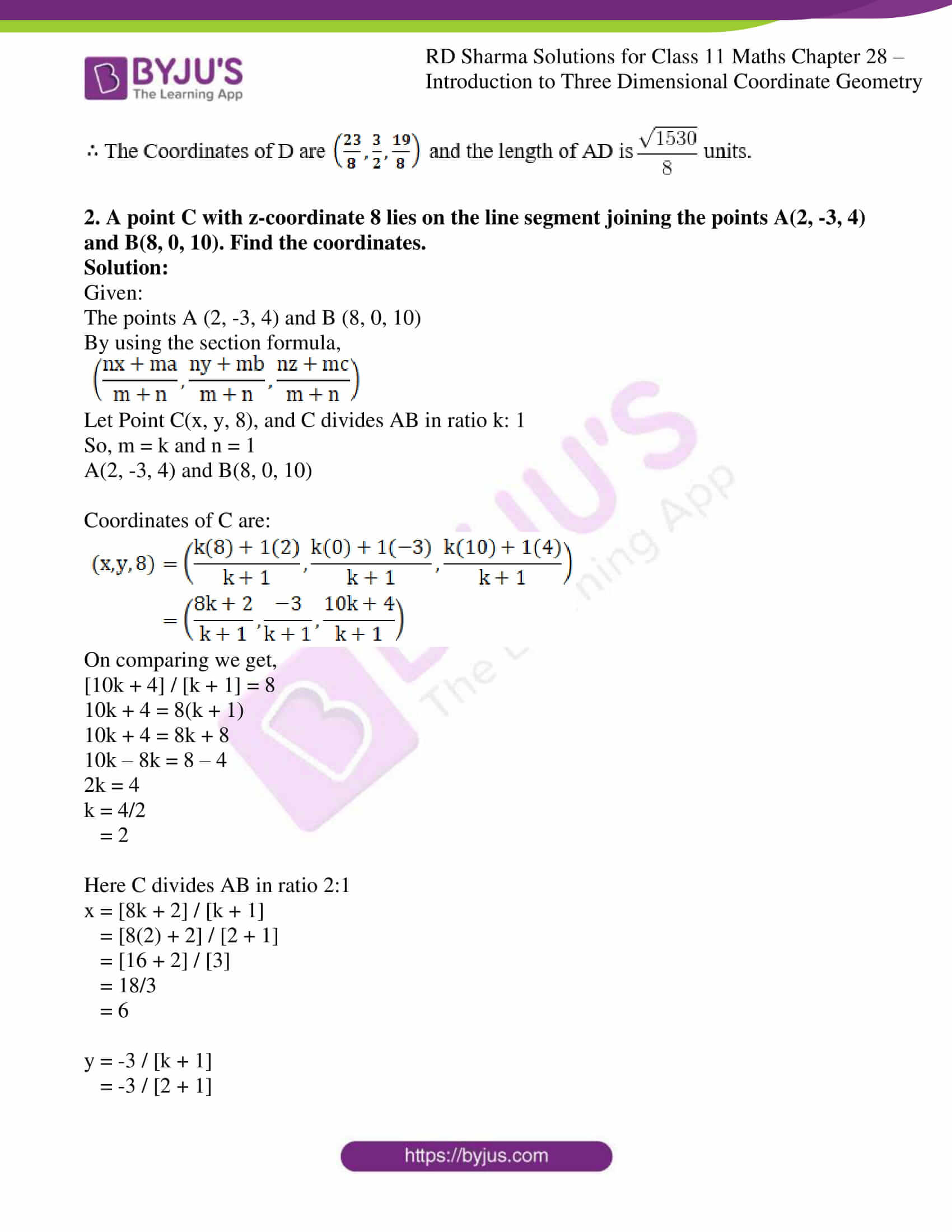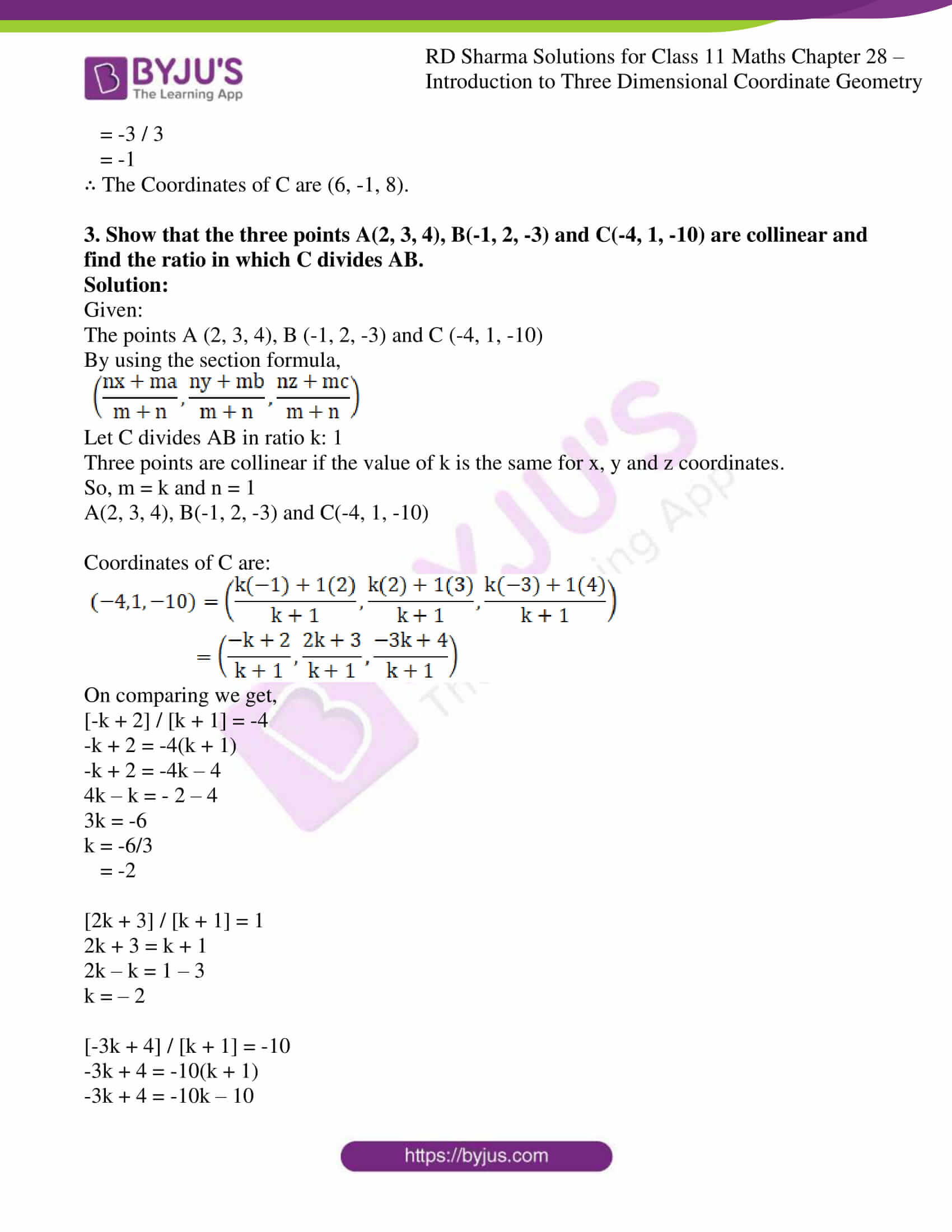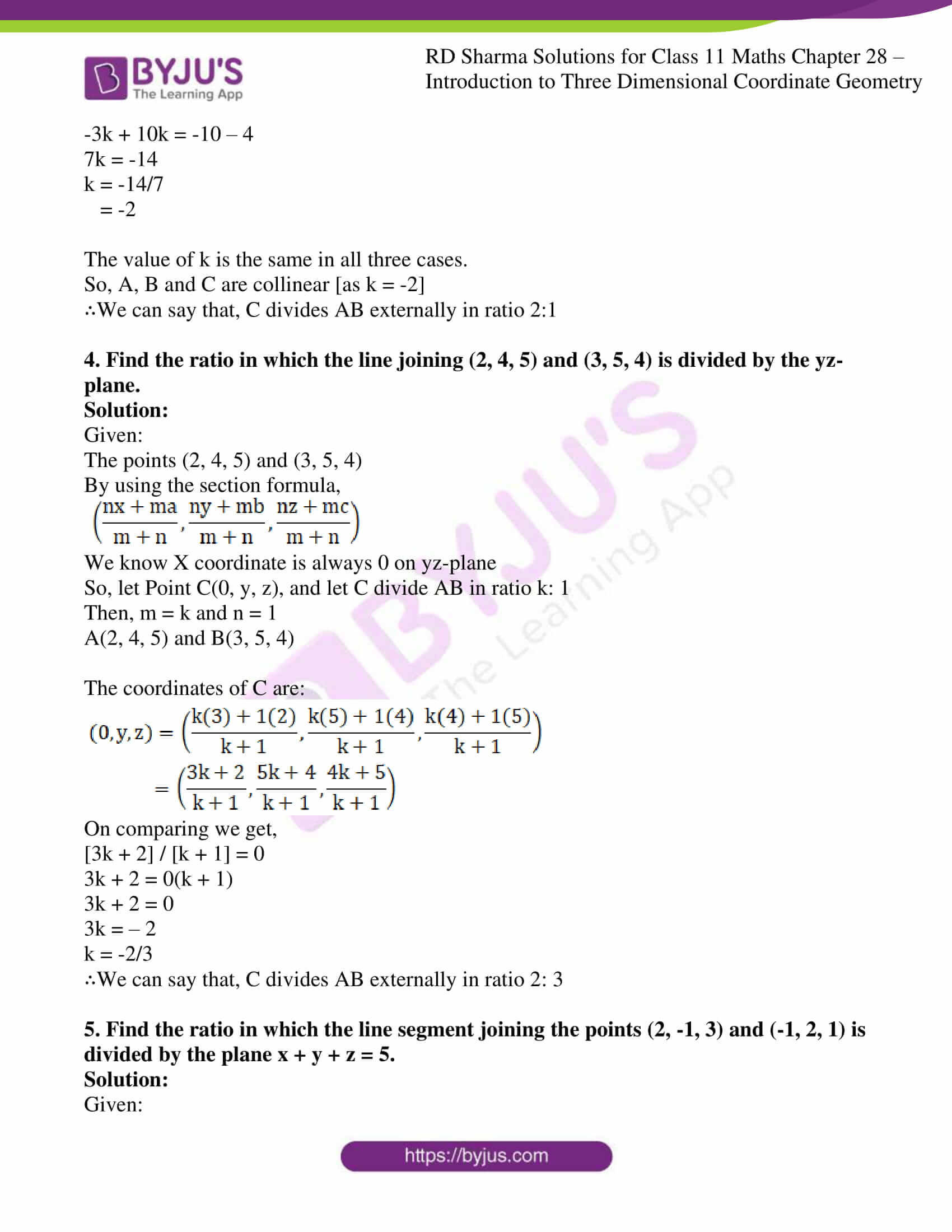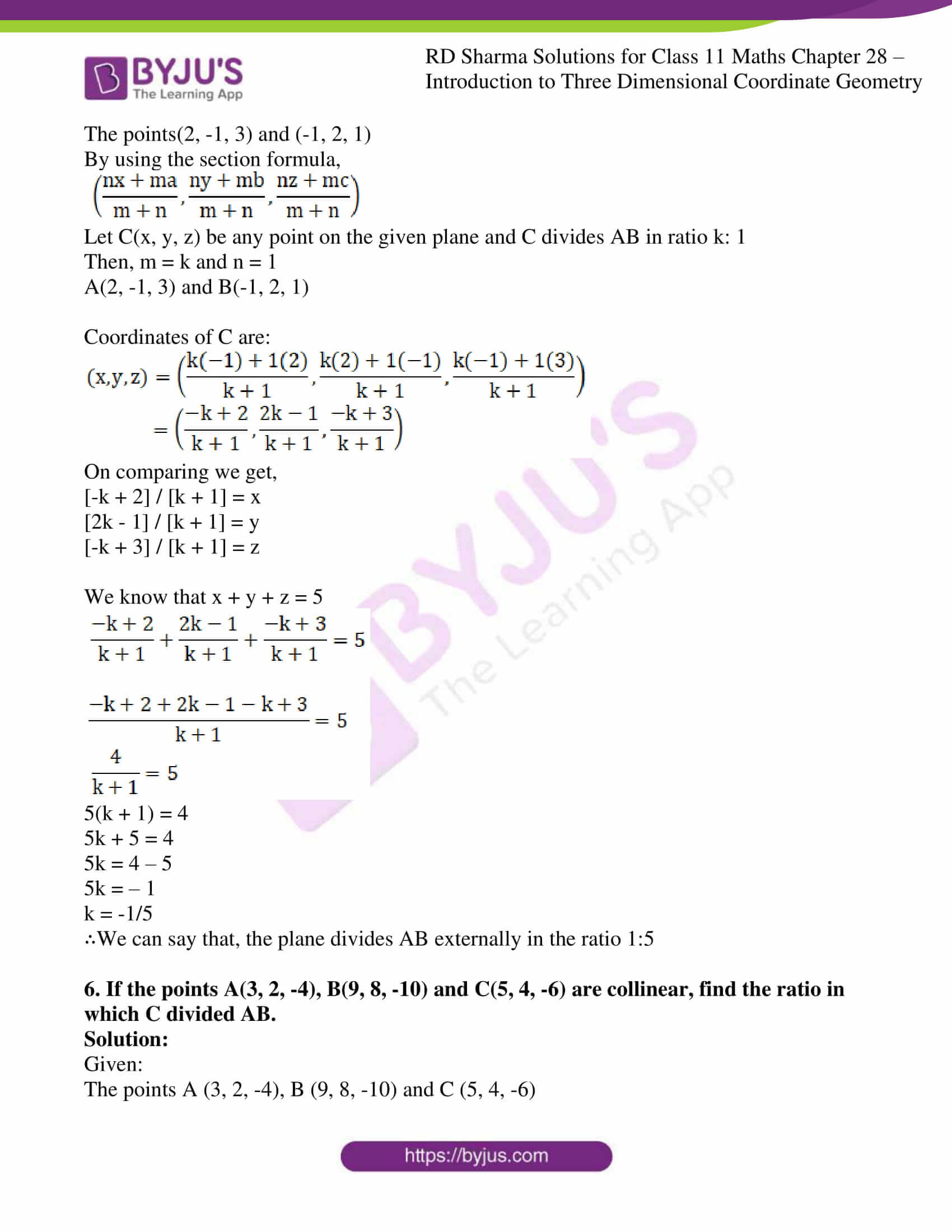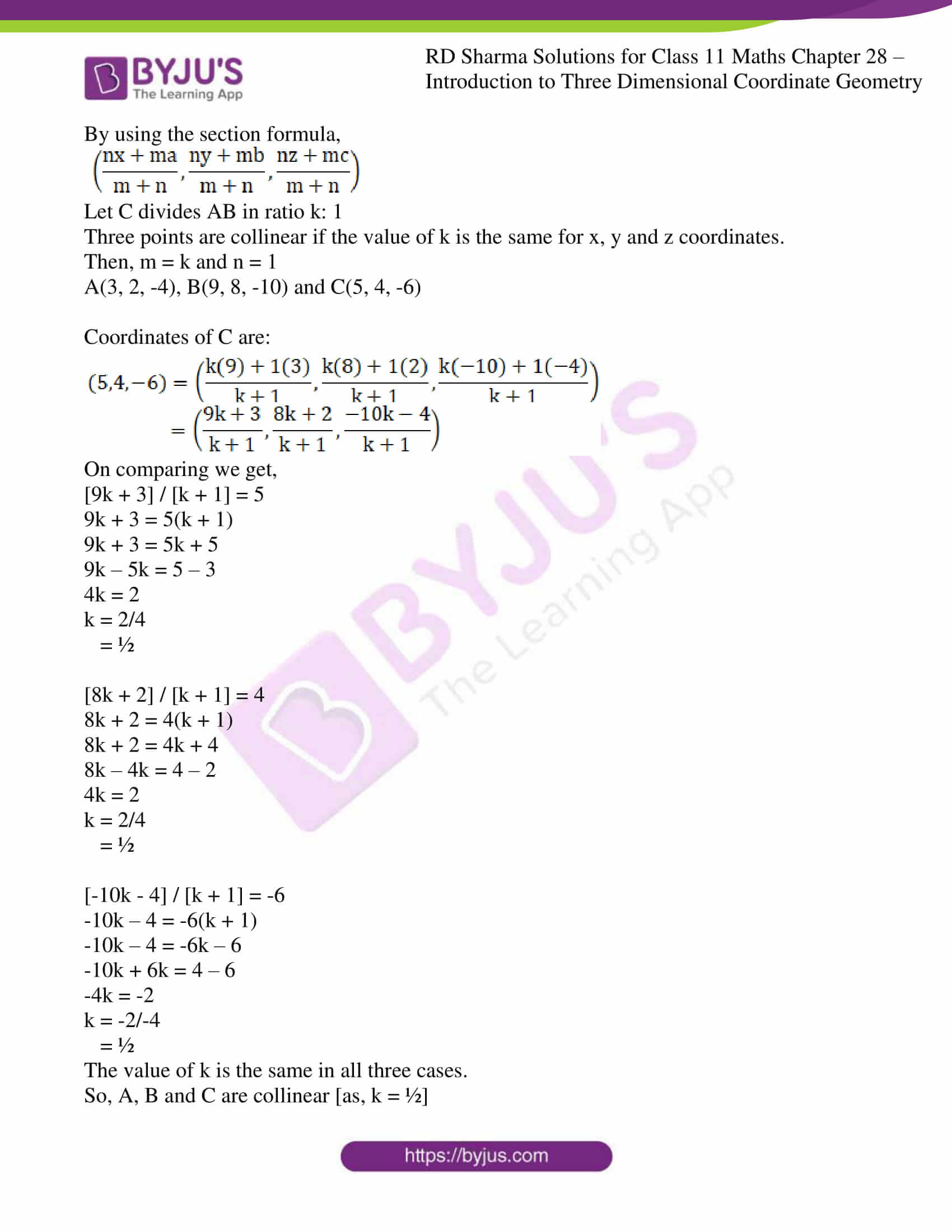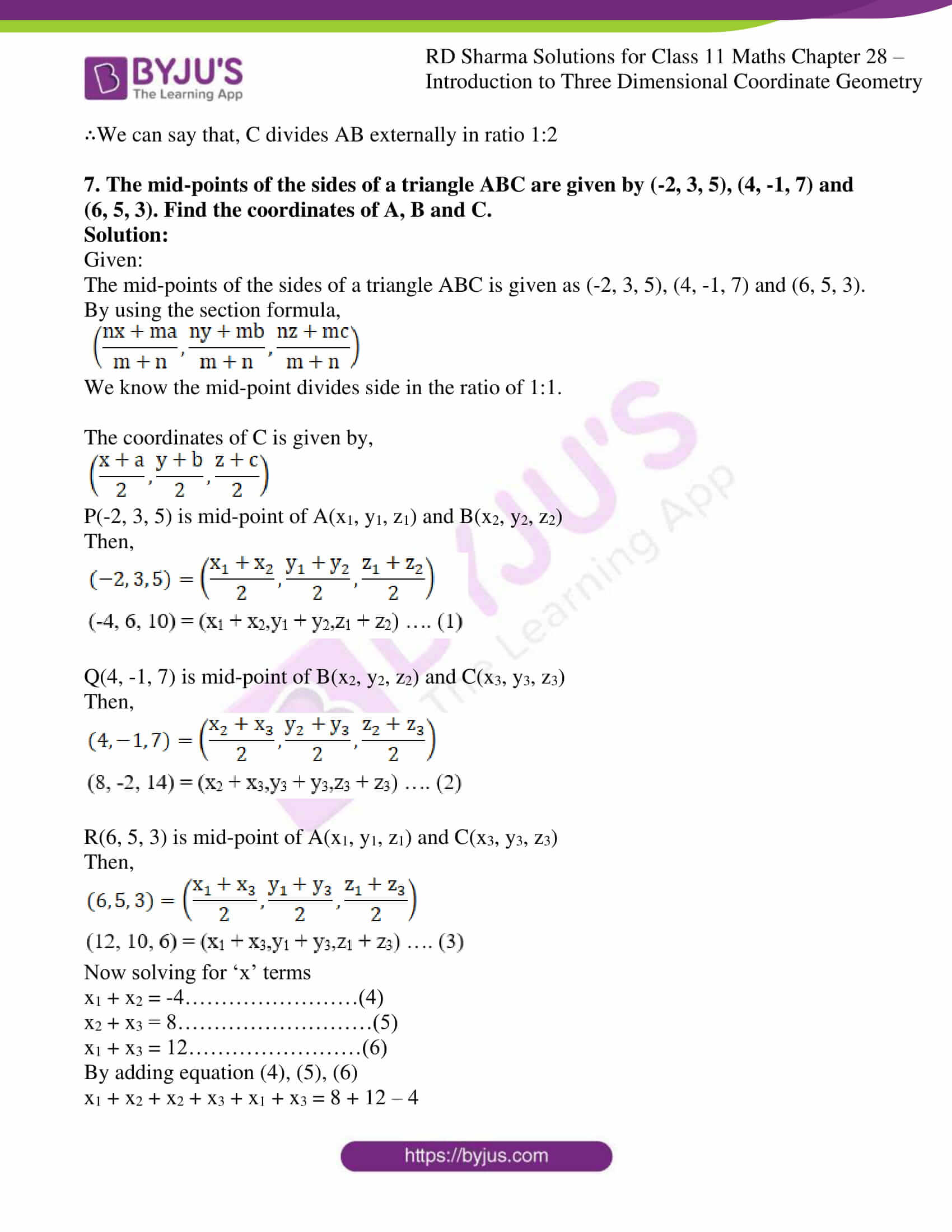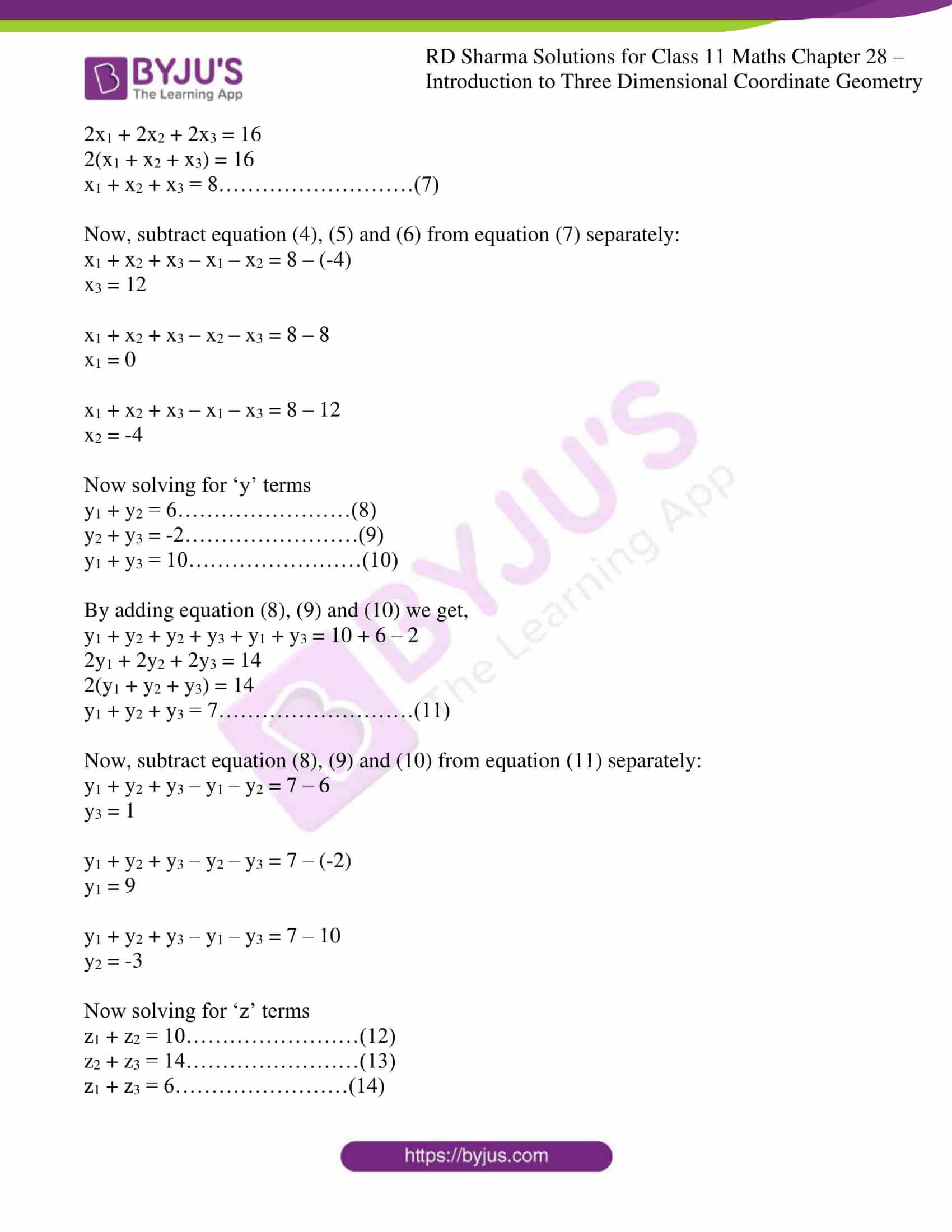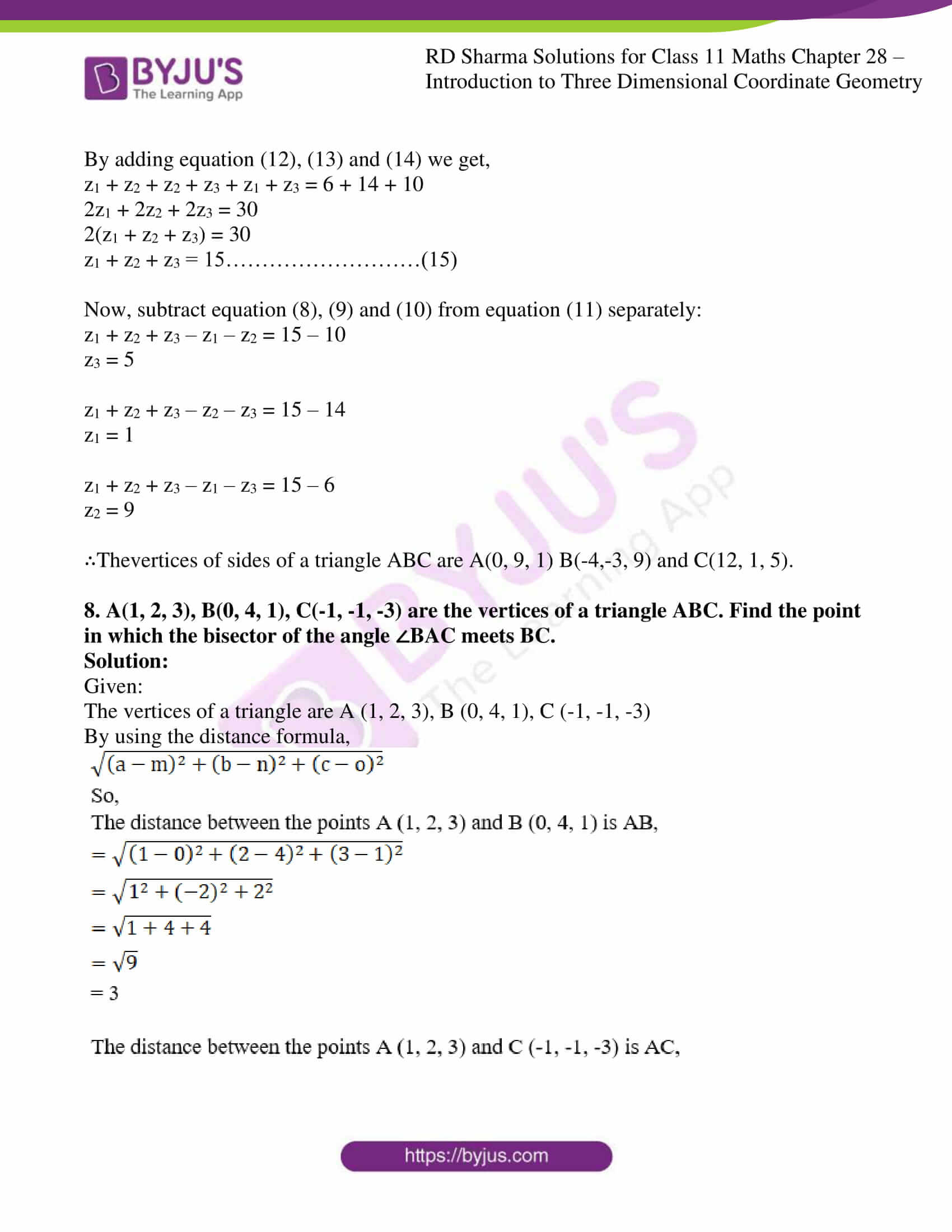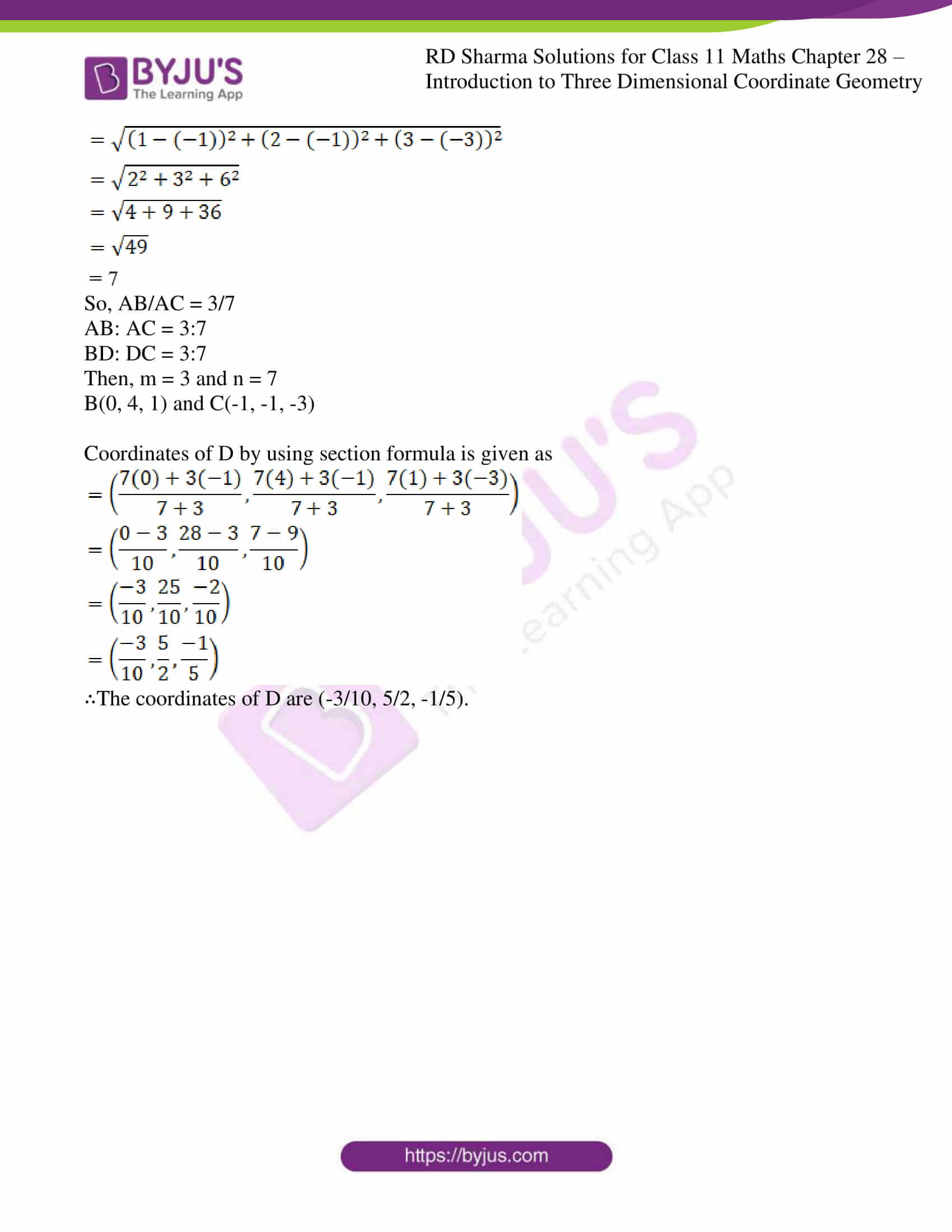### Access answers to RD Sharma Solutions for Class 11 Maths Exercise 28.3 Chapter 28 – Introduction to Three Dimensional Coordinate Geometry

#### ExERCISE 28.3 PAGE NO: 28.19

1. The vertices of the triangle are A(5, 4, 6), B(1, -1, 3) and C(4, 3, 2). The internal bisector of angle A meets BC at D. Find the coordinates of D and the length AD.

Solution:

Given:

The vertices of the triangle are A (5, 4, 6), B (1, -1, 3) and C (4, 3, 2).

By using the formulas let us find the coordinates of D and the length of AD

The distance between any two points (a, b, c) and (m, n, o) is given by,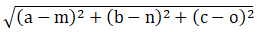The section formula is given as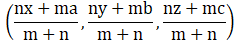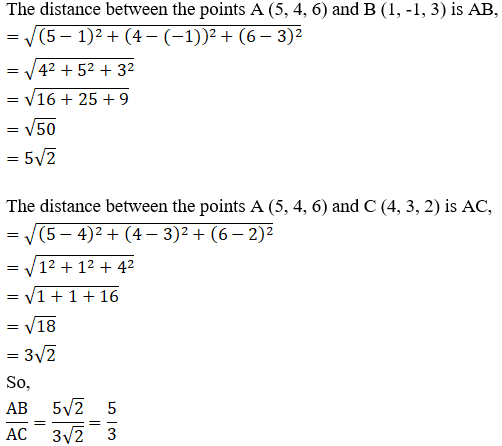AB : AC = 5:3

BD: DC = 5:3

So, m = 5 and n = 3

B(1, -1, 3) and C(4, 3, 2)

Coordinates of D using section formula: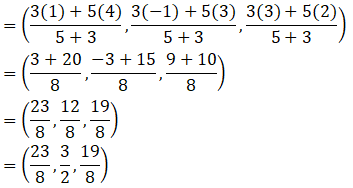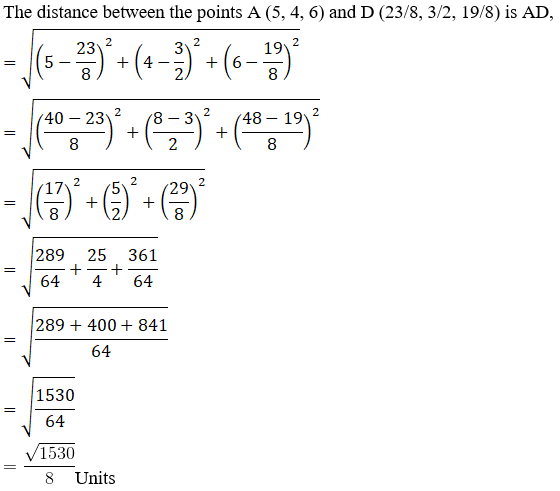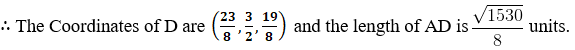2. A point C with z-coordinate 8 lies on the line segment joining the points A(2, -3, 4) and B(8, 0, 10). Find the coordinates.

Solution:

Given:

The points A (2, -3, 4) and B (8, 0, 10)

By using the section formula,Let Point C(x, y, 8), and C divides AB in ratio k: 1

So, m = k and n = 1

A(2, -3, 4) and B(8, 0, 10)

Coordinates of C are: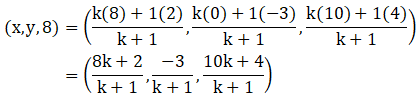On comparing we get,

[10k + 4] / [k + 1] = 8

10k + 4 = 8(k + 1)

10k + 4 = 8k + 8

10k – 8k = 8 – 4

2k = 4

k = 4/2

= 2

Here C divides AB in ratio 2:1

x = [8k + 2] / [k + 1]

= [8(2) + 2] / [2 + 1]

= [16 + 2] / 

= 18/3

= 6

y = -3 / [k + 1]

= -3 / [2 + 1]

= -3 / 3

= -1

∴ The Coordinates of C are (6, -1, 8).

3. Show that the three points A(2, 3, 4), B(-1, 2, -3) and C(-4, 1, -10) are collinear and find the ratio in which C divides AB.

Solution:

Given:

The points A (2, 3, 4), B (-1, 2, -3) and C (-4, 1, -10)

By using the section formula,Let C divides AB in ratio k: 1

Three points are collinear if the value of k is the same for x, y and z coordinates.

So, m = k and n = 1

A(2, 3, 4), B(-1, 2, -3) and C(-4, 1, -10)

Coordinates of C are: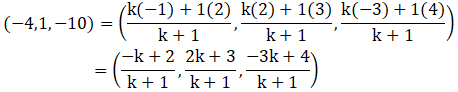On comparing we get,

[-k + 2] / [k + 1] = -4

-k + 2 = -4(k + 1)

-k + 2 = -4k – 4

4k – k = – 2 – 4

3k = -6

k = -6/3

= -2

[2k + 3] / [k + 1] = 1

2k + 3 = k + 1

2k – k = 1 – 3

k = – 2

[-3k + 4] / [k + 1] = -10

-3k + 4 = -10(k + 1)

-3k + 4 = -10k – 10

-3k + 10k = -10 – 4

7k = -14

k = -14/7

= -2

The value of k is the same in all three cases.

So, A, B and C are collinear [as k = -2]

∴We can say that, C divides AB externally in ratio 2:1

4. Find the ratio in which the line joining (2, 4, 5) and (3, 5, 4) is divided by the yz-plane.

Solution:

Given:

The points (2, 4, 5) and (3, 5, 4)

By using the section formula,We know X coordinate is always 0 on yz-plane

So, let Point C(0, y, z), and let C divide AB in ratio k: 1

Then, m = k and n = 1

A(2, 4, 5) and B(3, 5, 4)

The coordinates of C are: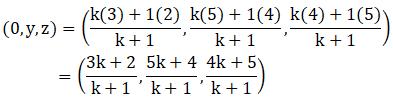On comparing we get,

[3k + 2] / [k + 1] = 0

3k + 2 = 0(k + 1)

3k + 2 = 0

3k = – 2

k = -2/3

∴We can say that, C divides AB externally in ratio 2: 3

5. Find the ratio in which the line segment joining the points (2, -1, 3) and (-1, 2, 1) is divided by the plane x + y + z = 5.

Solution:

Given:

The points(2, -1, 3) and (-1, 2, 1)

By using the section formula,Let C(x, y, z) be any point on the given plane and C divides AB in ratio k: 1

Then, m = k and n = 1

A(2, -1, 3) and B(-1, 2, 1)

Coordinates of C are: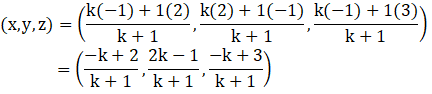On comparing we get,

[-k + 2] / [k + 1] = x

[2k – 1] / [k + 1] = y

[-k + 3] / [k + 1] = z

We know that x + y + z = 5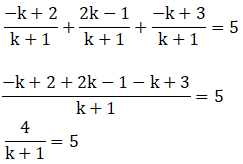5(k + 1) = 4

5k + 5 = 4

5k = 4 – 5

5k = – 1

k = -1/5

∴We can say that, the plane divides AB externally in the ratio 1:5

6. If the points A(3, 2, -4), B(9, 8, -10) and C(5, 4, -6) are collinear, find the ratio in which C divided AB.

Solution:

Given:

The points A (3, 2, -4), B (9, 8, -10) and C (5, 4, -6)

By using the section formula,Let C divides AB in ratio k: 1

Three points are collinear if the value of k is the same for x, y and z coordinates.

Then, m = k and n = 1

A(3, 2, -4), B(9, 8, -10) and C(5, 4, -6)

Coordinates of C are: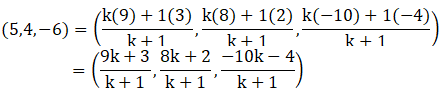On comparing we get,

[9k + 3] / [k + 1] = 5

9k + 3 = 5(k + 1)

9k + 3 = 5k + 5

9k – 5k = 5 – 3

4k = 2

k = 2/4

= ½

[8k + 2] / [k + 1] = 4

8k + 2 = 4(k + 1)

8k + 2 = 4k + 4

8k – 4k = 4 – 2

4k = 2

k = 2/4

= ½

[-10k – 4] / [k + 1] = -6

-10k – 4 = -6(k + 1)

-10k – 4 = -6k – 6

-10k + 6k = 4 – 6

-4k = -2

k = -2/-4

= ½

The value of k is the same in all three cases.

So, A, B and C are collinear [as, k = ½]

∴We can say that, C divides AB externally in ratio 1:2

7. The mid-points of the sides of a triangle ABC are given by (-2, 3, 5), (4, -1, 7) and (6, 5, 3). Find the coordinates of A, B and C.

Solution:

Given:

The mid-points of the sides of a triangle ABC is given as (-2, 3, 5), (4, -1, 7) and (6, 5, 3).

By using the section formula,We know the mid-point divides side in the ratio of 1:1.

The coordinates of C is given by,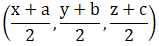P(-2, 3, 5) is mid-point of A(x1, y1, z1) and B(x2, y2, z2)

Then,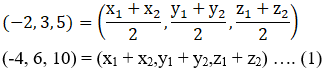Q(4, -1, 7) is mid-point of B(x2, y2, z2) and C(x3, y3, z3)

Then,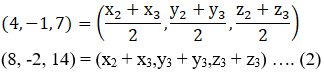R(6, 5, 3) is mid-point of A(x1, y1, z1) and C(x3, y3, z3)

Then,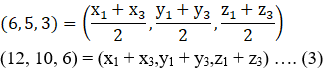Now solving for ‘x’ terms

x1 + x2 = -4……………………(4)

x2 + x3 = 8………………………(5)

x1 + x3 = 12……………………(6)

By adding equation (4), (5), (6)

x1 + x2 + x2 + x3 + x1 + x3 = 8 + 12 – 4

2x1 + 2x2 + 2x3 = 16

2(x1 + x2 + x3) = 16

x1 + x2 + x3 = 8………………………(7)

Now, subtract equation (4), (5) and (6) from equation (7) separately:

x1 + x2 + x3 – x1 – x2 = 8 – (-4)

x3 = 12

x1 + x2 + x3 – x2 – x3 = 8 – 8

x1 = 0

x1 + x2 + x3 – x1 – x3 = 8 – 12

x2 = -4

Now solving for ‘y’ terms

y1 + y2 = 6……………………(8)

y2 + y3 = -2……………………(9)

y1 + y3 = 10……………………(10)

By adding equation (8), (9) and (10) we get,

y1 + y2 + y2 + y3 + y1 + y3 = 10 + 6 – 2

2y1 + 2y2 + 2y3 = 14

2(y1 + y2 + y3) = 14

y1 + y2 + y3 = 7………………………(11)

Now, subtract equation (8), (9) and (10) from equation (11) separately:

y1 + y2 + y3 – y1 – y2 = 7 – 6

y3 = 1

y1 + y2 + y3 – y2 – y3 = 7 – (-2)

y1 = 9

y1 + y2 + y3 – y1 – y3 = 7 – 10

y2 = -3

Now solving for ‘z’ terms

z1 + z2 = 10……………………(12)

z2 + z3 = 14……………………(13)

z1 + z3 = 6……………………(14)

By adding equation (12), (13) and (14) we get,

z1 + z2 + z2 + z3 + z1 + z3 = 6 + 14 + 10

2z1 + 2z2 + 2z3 = 30

2(z1 + z2 + z3) = 30

z1 + z2 + z3 = 15………………………(15)

Now, subtract equation (8), (9) and (10) from equation (11) separately:

z1 + z2 + z3 – z1 – z2 = 15 – 10

z3 = 5

z1 + z2 + z3 – z2 – z3 = 15 – 14

z1 = 1

z1 + z2 + z3 – z1 – z3 = 15 – 6

z2 = 9

∴Thevertices of sides of a triangle ABC are A(0, 9, 1) B(-4,-3, 9) and C(12, 1, 5).

8. A(1, 2, 3), B(0, 4, 1), C(-1, -1, -3) are the vertices of a triangle ABC. Find the point in which the bisector of the angle ∠BAC meets BC.

Solution:

Given:

The vertices of a triangle are A (1, 2, 3), B (0, 4, 1), C (-1, -1, -3)

By using the distance formula,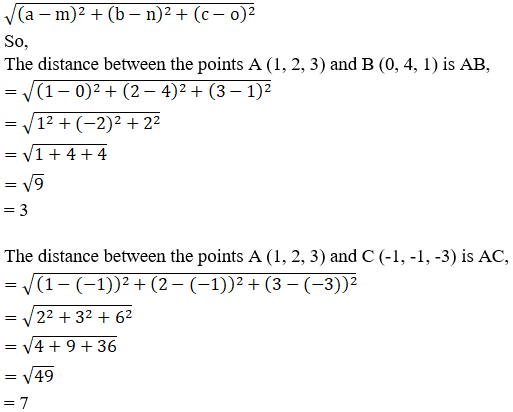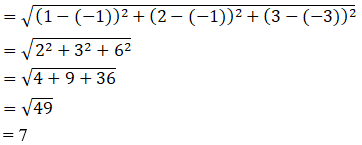So, AB/AC = 3/7

AB: AC = 3:7

BD: DC = 3:7

Then, m = 3 and n = 7

B(0, 4, 1) and C(-1, -1, -3)

Coordinates of D by using section formula is given as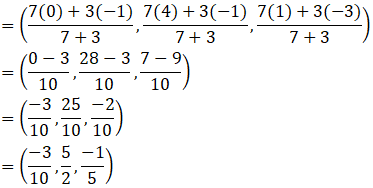∴The coordinates of D are (-3/10, 5/2, -1/5).